# Spearman’s Correlation

The correlation between two variables,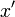$x'$ and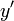$y'$ is: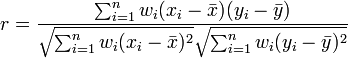$r = \frac{\sum ^n _{i=1}w_i(x_i - \bar{x})(y_i - \bar{y})}{\sqrt{\sum ^n _{i=1}w_i(x_i - \bar{x})^2} \sqrt{\sum ^n _{i=1}w_i(y_i - \bar{y})^2}}$

where: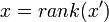$x = rank(x')$ and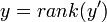$y = rank(y')$ where ties are replaced by their means,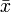$\bar{x}$ and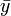$\bar{y}$ are their means, respectively,$w_i$ is the Calibrated Weight for the$i$th of$n$ observations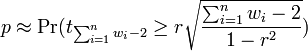$p \approx \Pr(t_{\sum^n_{i=1}w_i-2} \ge r\sqrt{\frac{\sum^n_{i=1}w_i-2}{1 - r^2}})$

Note: a bug existed in versions of Q prior to Q5.0.2, caused by Spearman ranks being calculated without omitting the cases with missing values in any of the two input variables. The impact of this bug should be minimal and proportional to the amount of missing values in the data set. We have observed that the bug typically resulted in a difference in the reported Spearman correlation at the 4th decimal place compared to the correct value. As a result, is unlikely that this bug would have affected Spearman correlation significance test results and any inferences made from them.